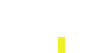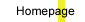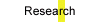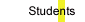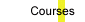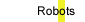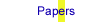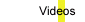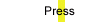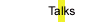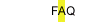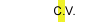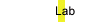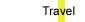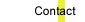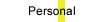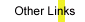## THEORETICAL MODELS OF LEARNING TO LEARN

by Jonathan Baxter

A Machine can only learn if it is biased in some way. Typically the bias is supplied by hand, for example through the choice of an appropriate set of features. However, if the learning machine is embedded within an environment of related tasks, then it can learn its own bias by learning sufficiently many tasks from the environment. In this paper two models of bias learning (or equivalently, learning to learn) are introduced and the main theoretical results presented. The first model is a PAC-type model based on empirical process theory, while the second is a hierarchical Bayes model.